# Algorithmic worlds

## -

### Search blog posts

Date:

Tags:

Next posts

2013-06-09 Variations on Nova

2013-05-12 Two new gigapixel images

2013-04-28 Lattes Julia sets

2012-10-21 3d hyperbolic limit sets

2012-07-01 Fractal sea creatures

2012-06-24 Nicholas A. Cope

2012-06-16 3d printed Julia set

2012-05-06 Pep Ventosa

2012-04-06 Fractal automata

2012-03-30 Periodic Julia patterns

# Blog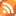A blog about algorithmic art and fractal aesthetic. Click here to subscribe to the RSS feed.

March 30th 2012

## Periodic Julia patterns

This blog post is a continuation of the previous post. There, we had a look at the behavior of two dense fractal patterns under conformal transformations. It was obvious that the general appearance of the dense Julia pattern was left unchanged, while the fractal Mondrian pattern was badly deformed. We saw that the conformal transformation obtained by the complex sine, when the plane is seen as the complex numbers, had the nice property of yielding a pattern periodic in one direction. The question that was asked at the end of the post was whether there was a conformal transformation that would produce a pattern periodic in two directions.

Let us start with a dumb way of trying to solve the problem. We might say, well, we know that the real sine is periodic, so why not combine two real sines applied to the real and imaginary axis? The transform would look like (x,y) -> (sin(x),sin(y)). The trouble with this is that the resulting transformation is not at all conformal. This is a good occasion to show that dense Julia patterns are badly distorted by non-conformal transformations. Here is the original pattern, together with the deformed pattern.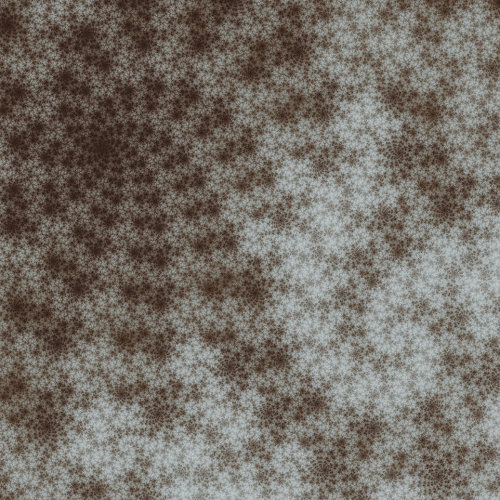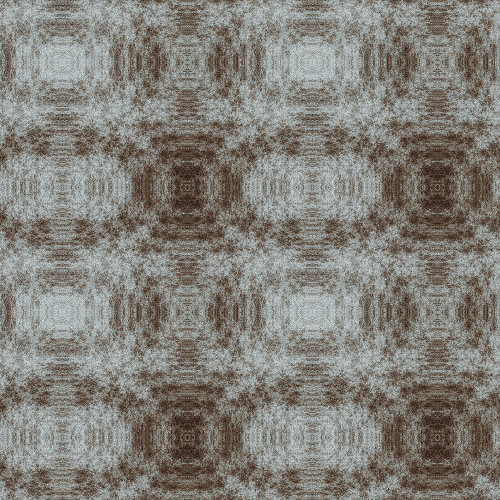Above, a dense Julia pattern. Below, the same pattern after the application of the transformation (x,y) -> (sin(x),sin(y)).

Clearly, there is a striking difference compared to its behavior under conformal transformation discussed in the previous blog post. Just for fun, here is what happens to the fractal Mondrian pattern: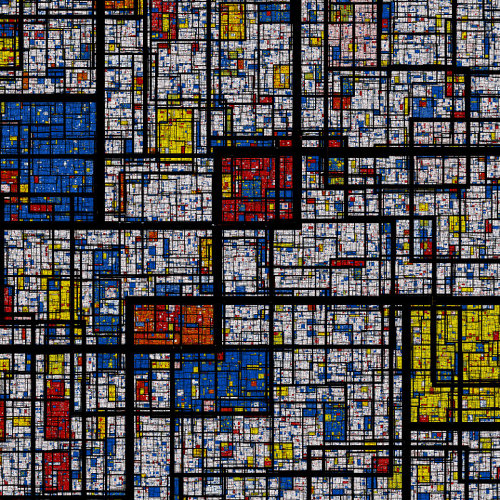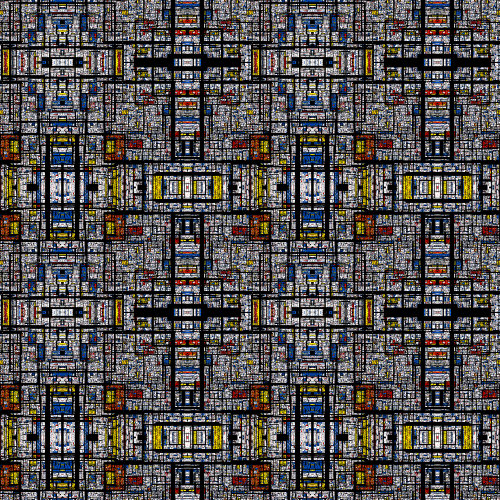Above, a fractal Mondrian pattern. Below, the same pattern after the application of the transformation (x,y) -> (sin(x),sin(y)).

Interestingly, the fractal Mondrian pattern does not seem too distorted, probably because the transformation maps vertical lines to vertical lines, and horizontal lines to horizontal lines. At any rate, this little experiment shows that this is clearly not the way to obtain something we could call a periodic Julia pattern. We really need to find a conformal transformation which is periodic on the plane.

Even if in hindsight, the solution to this problem turns out to be quite obvious, I couldn't have found it without a nice little geometrical reasoning that I will sketch here. For this, we need the notion of a covering. If you're a girl with long hair, you might sometimes ties them in a tail with an elastic. To do this, you're alternately twisting the elastic and pulling the tail through it. Once you're done, the elastic is wrapped several times around the tail. Geometrizing the situation, we have a circle (the elastic) wrapped several times around a circle (a section of the tail). That's the most basic example of a covering. More generally, a covering is a continuous map from a space (the cover) to another (the base) such that the preimage of any point in the base is formed by n disjoind points of the cover, for a fixed given n, called the order of the covering. In our example, both the base and the cover are circles, and the order is the number of times the elastic is wrapped around the tail. There is a slight generalization of the notion of covering, a branched covering, where the condition on the preimage is true everywhere but at a finite number of points (the branch points). Interestingly, there is an order 2 covering of the sphere by a torus, with four branch points. I do not know of any very elementary way of visualizing this map. The details are explained in many references, for instance in this one, section 5, example 3.

Why are we interested in tori and spheres? First, the Julia patterns naturally live on the sphere. On the other hand, any periodic pattern on the plane can be seen as a decoration of a torus. Indeed, a torus is a product of two circles. These two circles can be parameterized by two (periodic) angles. Therefore any decoration of the torus can be mapped to a periodic pattern on the plane. (And actually, this is another example of a covering, this time of infinite order). Now as we have a decoration of the sphere given by the dense Julia pattern, we can "pull back" this decoration from the sphere to the torus, and then back on the plane, to get a periodic dense Julia pattern on the plane. The "pull back" operation means the following. Take any point of the plane. Map it to the torus, and then on the sphere by the maps mentioned above. Color the point the same color as the point of the Julia pattern you hit on the sphere. The crucial point in this story is that all the maps can be taken to be conformal, so we do get the periodic conformal map we were after.

The explicit form of this map is given by the Weierstrass P-function. One can guess that it is a periodic function just by looking at it, as it involves essentially the sum over a lattice of a single summand (with some regularization). It is also clear that it yields a conformal transformation, because it is meromorphic.

Now it's time to see what the resulting patterns look like in pictures. Let us start with the fractal Mondrian pattern.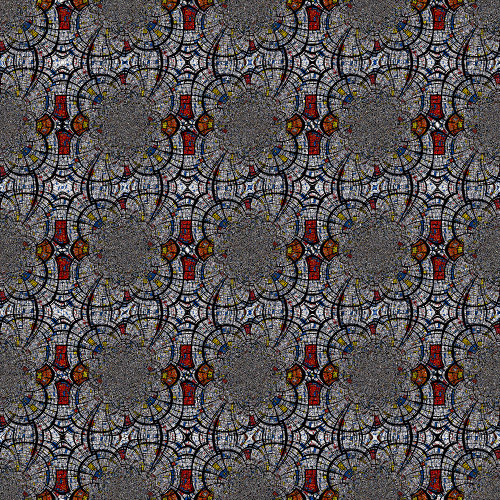A fractal Mondrian pattern deformed by the Weierstrass P-function.

As expected, the pattern is barely recognizable. Around the poles of the P-function, the fractal Mondrian pattern becomes very small and appears in the form of gray dust. Compare this with the dense Julia pattern: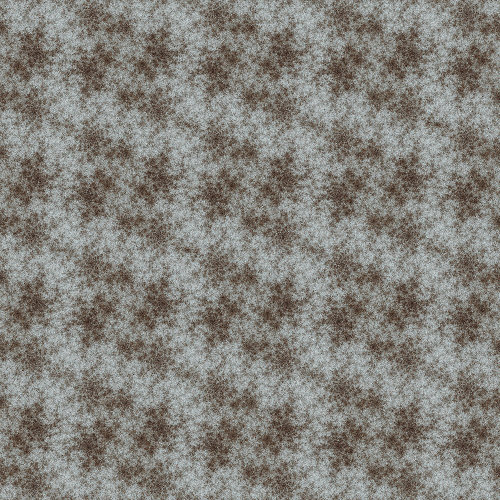A periodic dense Julia pattern, obtained by deformation with the Weierstrass P-function. Click for a highher resolution image.

The patterns is periodic, but still displays its characteristic shapes. Here is another one, based on 20111029-1: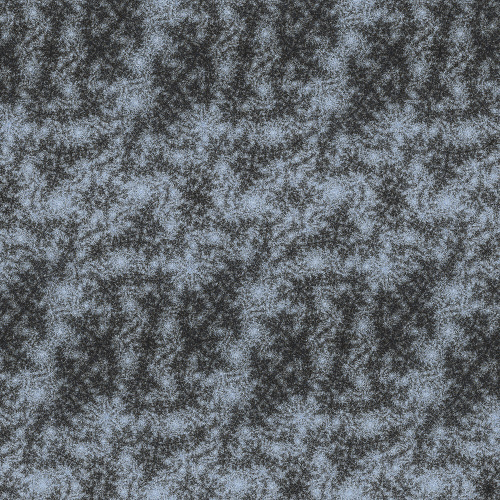A periodic dense Julia pattern, obtained by deformation with the Weierstrass P-function. Click for a highher resolution image.

I find these periodic dense Julia patterns quite beautiful. Here are a few more, used as background html pictures:
20120227
20120228
20120302
20120303
20120315
.

An animation featuring a dense Julia pattern is also rendering... and should be done in about two months. :-\

[Update:] Here it is!